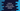# How to use Python string partition method## How to use Python string partition method:

The string partition method is used to divide a string in two parts in Python. This is a quick way to break a string in Python. It takes separator as its parameter and splits the string at this separator.

Let’s learn how it works with examples.

### Definition of partition:

The definition of partition method is:

``str.partition(s)``

Here,

• str is the string
• s is the separator to split the string. This argument is a required argument.

#### Return value of partition:

The partition method returns a tuple that holds three elements. It returns the string part before the separator as the first value, the separator itself as the second value and the remaining part as the third value.

It uses the first occurrence of the separator. If the separator is found multiple times, it ignores the others.

Let’s try it with different examples:

### Example 1: partition with one separator:

Let’s take a look at the below example:

``````given_str = 'Hello @ World !!'

print(given_str.partition('@'))``````

In this example, we are passing @ to the partition method. @ appears only once in the string given_str. It will return:

``('Hello ', '@', ' World !!')``

As you can see here, in the tuple the substring before @ is placed at the first position, the substring after @ is placed at the third position and @ is in the middle. Note that blank spaces are not removed from the words.

### Example 2: partition with multiple separators:

If we have multiple occurrences of the separator, it will consider only the first one and ignore the rest. For example,

``````given_str = 'Hello @ World @ Hello!!'

print(given_str.partition('@'))``````

It will print:

``('Hello ', '@', ' World @ Hello!!')``

### Example 3: Case sensitive separator:

partition is case-sensitive, i.e. the separator we are passing is case sensitive. For example,

``````given_str = 'Hello a A World'

print(given_str.partition('A'))``````

Here, the string has a and A, both characters. But, we are passing A to partition. It will print:

``('Hello a ', 'A', ' World')``

### Example 4: Word separator:

Let’s try it with a word. It works in a similar way:

``````given_str = 'Hello And World'

print(given_str.partition('And'))``````

It will print:

``('Hello ', 'And', ' World')``

### Example 5: Empty separator:

If we pass an empty string as the separator, it throws a ValueError.

``````given_str = 'Hello And World'

print(given_str.partition(''))``````

It will throw a ValueError:

``````Traceback (most recent call last):
File "/code/python/example.py", line 3, in <module>
print(given_str.partition(''))
ValueError: empty separator``````